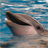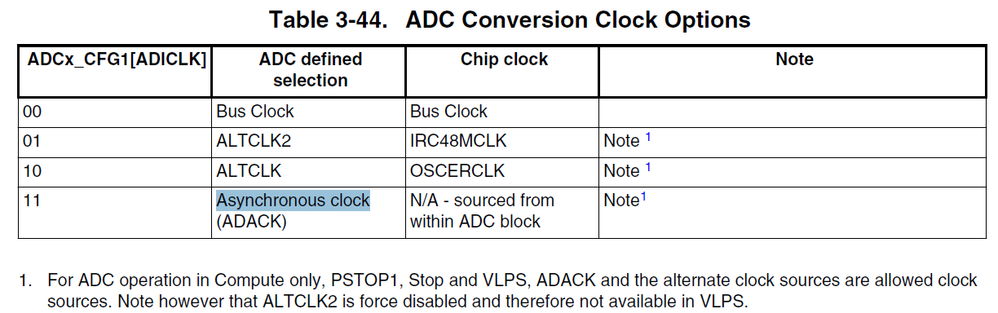# How to calculate the ADC Clock?

cancel
Showing results for
Did you mean:

## How to calculate the ADC Clock?

2,373 ViewsContributor I

Hello, I'm trying use ADC at FRDM-K22F, it's working, but I didn't get success on calculate the ADC clock. I'm using http://www.nxp.com/products/microcontrollers-and-processors/arm-processors/kinetis-cortex-m-mcus/adc... for calculate the ADC clock, but the result the calculator don't is equal at result of conversion.

I'm setting my ADC clock this way:

What's the result of ADC clock for this example?

Thanks.

Labels (1)
• ### General

2 Replies
1,314 ViewsNXP TechSupport

Regarding your question about the ADC clock frequency, from hardware perspective, I suggest you should check the following register setting.

For K22, I copied the part here:2)there is divider set up in the ADCx_CFG[ADIV], I copy it here.

Clock Divide Select

Selects the divide ratio used by the ADC to generate the internal clock ADCK.

00 The divide ratio is 1 and the clock rate is input clock.

01 The divide ratio is 2 and the clock rate is (input clock)/2.

10 The divide ratio is 4 and the clock rate is (input clock)/4.

11 The divide ratio is 8 and the clock rate is (input clock)/8.

For your code, I think you select bus clock as ADC clock source with ADICLK bit=00 in binary, the divider is 8 with ADIV bits=11 in binary, the ADC clock frequency is (bus clock)/8. I do not know your bus clock frequency, it is dependent on the MCG mode, and SIM_CLKDIV1 register setting.

B R

Xiangjun Rong

1,314 ViewsContributor I

Hi Xiangjun,

My bus clock is 60 MHz divided for 8, so, my ADC Clock is 7,5 MHz. Now, I want find the conversion time per sample, I have to use this formula  (ConversionTime = SFCAdder + AVGNUM x (BCT + LSTAdder + HSCAdder)) ?

Thank you very much.